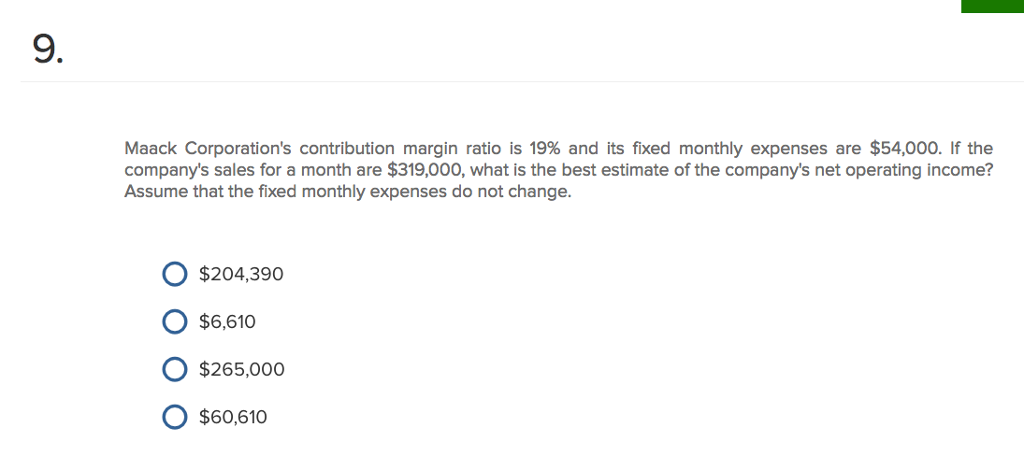# Question & Answer: Maack Corporation's contribution margin ratio is 19% and its fixed monthly expenses are \$54,000. If the com…..Maack Corporation’s contribution margin ratio is 19% and its fixed monthly expenses are \$54,000. If the company’s sales for a month are \$319,000, what is the best estimate of the company’s net operating income? Assume that the fixed monthly expenses do not change. \$204.390 \$6, 610 \$265.000 \$60, 610

Don't use plagiarized sources. Get Your Custom Essay on
Question & Answer: Maack Corporation's contribution margin ratio is 19% and its fixed monthly expenses are \$54,000. If the com…..
GET AN ESSAY WRITTEN FOR YOU FROM AS LOW AS \$13/PAGE

The correct answer would be \$6,610

Net Operaing income = (Contribution margin ratio * Sales)- Fixed expenses

= (19%*\$319,000)-\$54,000

= \$60,610-\$54,000

= \$6,610